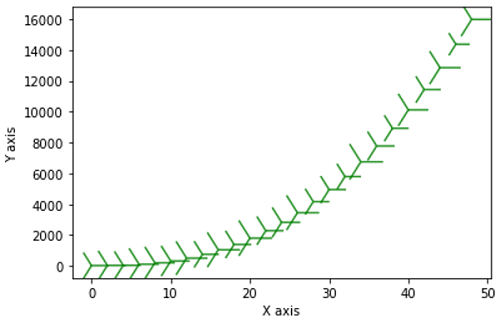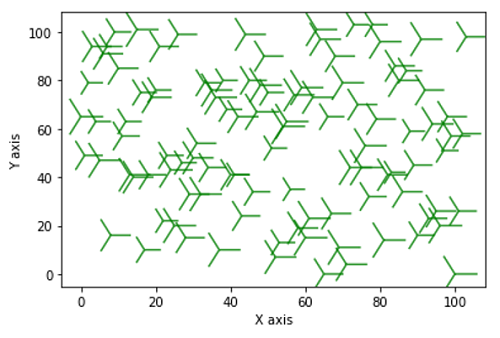# Python | Y Shape Scatter Marker

In this tutorial, we are going to learn how to use Y shape scatter marker in scatter plot using matplotlib in Python?
Submitted by Anuj Singh, on August 17, 2020

There are few markers that can be used everywhere such as circular or square markers. But, matplotlib has some other inbuilt defined markers such as tri-edge Y shape marker which is used in directional plots. In matplotlib.pyplot command marker='4' for our desired marker style and the following figure illustrates the example of the same.

Illustrations:## Python code for y-shape scatter marker

```import matplotlib.pyplot as plt
import numpy as np

x = np.arange(0.0, 50.0, 2.0)
y = x ** 2.5 + np.random.rand(*x.shape) * 30.0
s = np.random.rand(*x.shape) * 800 + 500

plt.scatter(x, y, s, c="g", alpha=0.8, marker='4')
plt.xlabel("X axis")
plt.ylabel("Y axis")
plt.show()

x = np.arange(104)
y = np.random.randint(0,104,104)
s = np.random.rand(104) * 800 + 500

plt.scatter(x, y, s, c="g", alpha=0.8, marker='4')
plt.xlabel("X axis")
plt.ylabel("Y axis")
plt.show()
```

Output:

```Output is as Figure
```

Languages: » C » C++ » C++ STL » Java » Data Structure » C#.Net » Android » Kotlin » SQL
Web Technologies: » PHP » Python » JavaScript » CSS » Ajax » Node.js » Web programming/HTML
Solved programs: » C » C++ » DS » Java » C#
Aptitude que. & ans.: » C » C++ » Java » DBMS
Interview que. & ans.: » C » Embedded C » Java » SEO » HR
CS Subjects: » CS Basics » O.S. » Networks » DBMS » Embedded Systems » Cloud Computing
» Machine learning » CS Organizations » Linux » DOS
More: » Articles » Puzzles » News/Updates